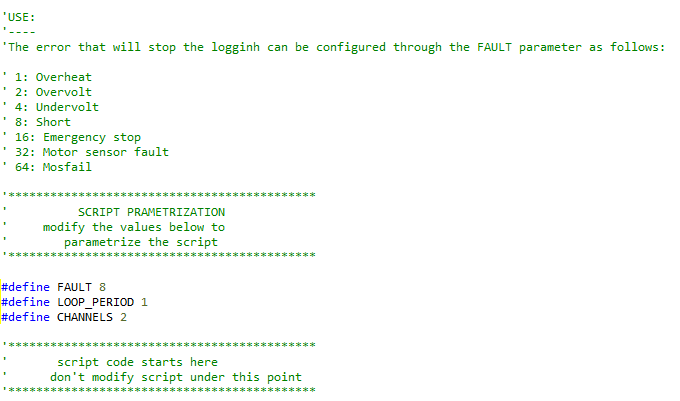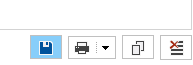Sometimes an error is hard to be reproduced or its occurrence seems completely random, so the necessary logs for troubleshooting cannot be recorded easily. the below script can be used to take some debugging logs until a fault has occurred.

The user just need to configure on the script the exact error we is willing to capture through the FAULT as follows:The number of channels must be configured as well. Use 2 for dual channel controller. Then  run the script and stay at the console tab until the error has been reproduced. Copy the logs and send to a FAE for troubleshooting.It is important to be on console tab until the error has been replicated to acquire adequate data. If the system is not being controlled by a master device, serial commands can be given from console tab to run the motors. The watchdog timer must have a respective value to achieve that.

The script follows:

````'******************************************`
`'         LOG UNTIL FAULT SCRIPT`
`'******************************************`

`option explicit`

`'DISCLAIMER:`
`'----------`

`'The script is tested and validated by Roboteq and is believed to be fault-free. `
`'The possibility always exists, however, that the particular configuration and/or use condition uncovers a fault `
`'that escaped our validation test coverage. Always extensively test the script under your use conditions prior `
`'to deploying it in the field.`

`'PURPOSE:`
`'--------`
`'This script can be used for cases that a fault cannot be captured easily `
`'and the user is not able to stop the logging process immediately after the fault.`
`'The script will plot the values in colsole and stop printing once the fault has occurred`
`'So the user will have the timeto copy the logs from console without them being overwritten`
`'The script can be configured to log until a specific error.`

`'USE:`
`'----`
`'The error that will stop the logginh can be configured through the FAULT parameter as follows:`

`' 1: Overheat`
`' 2: Overvolt`
`' 4: Undervolt`
`' 8: Short`
`' 16: Emergency stop`
`' 32: Motor sensor fault`
`' 64: Mosfail`

`'********************************************`
`'          SCRIPT PRAMETRIZATION`
`'     modify the values below to`
`'        parametrize the script`
`'********************************************`

`#define ``FAULT ``8  ``' Define the fault that will stop the logging process. See on the use section how to conifugre the fault`
`#define ``LOOP_PERIOD ``2 ``' this value is in millisecond. The console can hold maximum of 1000 lines, so total amount on logging will depend on the loop period`
`#define ``CHANNELS ``2 ``'the number of channels used`

`'********************************************`
`'       script code starts here`
`'      don't modify script under this point`
`'********************************************`

`dim ``rampedCommand`[`4`] `as integer`
`dim ``feedback`[`2`] `as integer`
`dim ``power`[`2`] `as integer`
`dim ``pha1`[`2`] `as integer`
`dim ``pha2`[`2`] `as integer`
`dim ``amps`[`2`] `as integer`
`dim ``batamps`[`2`] `as integer`
`dim ``temp`[`3`] `as integer`
`dim ``volts`[`3`] `as integer`

`dim ``channel ``as integer`

`start:`

`if `(`FAULT `= `1`)
`    ``gosub ``Overheat`
`elseif `(`FAULT`=`2`)
`    ``gosub ``Overvolt`
`elseif `(`FAULT`=`4`)
`    ``gosub ``Undervolt`
`elseif `(`FAULT`=`8`)
`    ``gosub ``Short`
`elseif `(`FAULT`=`16`)
`    ``'gosub Emergency`
`elseif `(`FAULT`=`32`)
`    ``'gosub Sensor`
`elseif `(`FAULT`=`64`)
`    ``'gosub Mosfail`
`end if`

`if`(`getvalue`(`_FF`,`1`) `and ``FAULT`)
`    ``terminate`
`end if`

`wait`(`LOOP_PERIOD`)

`goto ``start`

`'-----------------------------------------`
`'            Overheat routine`
`'-----------------------------------------`

`overheat:`

`for ``channel `= `1 ``andwhile ``channel `< (`CHANNELS`+`1`)
`    `
`    ``rampedCommand`[`channel``-1`] = `getvalue`(`_RMP`,`channel`)
`    ``feedback`[`channel``-1`] = `getvalue`(`_F`,`channel`)
`    ``power`[`channel``-1`] = `getvalue`(`_P`,`channel`)
`    ``amps`[`channel``-1`] = `getvalue`(`_A`,`channel`)
`    `
`    ``print`(`rampedCommand`[`channel``-1`], `"\t"`,`feedback`[`channel``-1`], `"\t"`,`power`[`channel``-1`], `"\t"`,`amps`[`channel``-1`], `"\t"`)

`next    `

`for ``channel `= `1 ``andwhile ``channel `< (`CHANNELS`+`2`)
`    `
`    ``temp`[`channel``-1`] = `getvalue`(`_T`,`channel`)
`    ``print`(`temp`[`channel``-1`],`"\t"`)
`    `
`next`

`print`(`" \n"`)

`return`

`'-----------------------------------------`
`'            Overvolt routine`
`'-----------------------------------------`

`overvolt:`

`for ``channel `= `1 ``andwhile ``channel `< (`CHANNELS`+`1`)
`    `
`    ``rampedCommand`[`channel``-1`] = `getvalue`(`_RMP`,`channel`)
`    ``feedback`[`channel``-1`] = `getvalue`(`_F`,`channel`)
`    ``power`[`channel``-1`] = `getvalue`(`_P`,`channel`)
`    ``amps`[`channel``-1`] = `getvalue`(`_A`,`channel`)
`    ``batamps`[`channel``-1`] = `getvalue`(`_BA`,`channel`)
`    `
`    ``print`(`rampedCommand`[`channel``-1`], `"\t"`,`feedback`[`channel``-1`], `"\t"`,`power`[`channel``-1`], `"\t"`,`amps`[`channel``-1`], `"\t"`,`batamps`[`channel``-1`], `"\t"`)

`next    `
`    `
`    ``volts`[`1`] = `getvalue`(`_V`,`2`)
`    ``print`(`volts`[`1`],`"\n"`)
`    `
`return`
` `
`'-----------------------------------------`
`'            Undervolt routine`
`'-----------------------------------------`

`undervolt:`

`for ``channel `= `1 ``andwhile ``channel `< (`CHANNELS`+`1`)
`    `
`    ``rampedCommand`[`channel``-1`] = `getvalue`(`_RMP`,`channel`)
`    ``feedback`[`channel``-1`] = `getvalue`(`_F`,`channel`)
`    ``power`[`channel``-1`] = `getvalue`(`_P`,`channel`)
`    ``amps`[`channel``-1`] = `getvalue`(`_A`,`channel`)
`    ``batamps`[`channel``-1`] = `getvalue`(`_BA`,`channel`)
`    ``volts`[`channel``-1`] = `getvalue`(`_V`,`channel`)
`    `
`    ``print`(`rampedCommand`[`channel``-1`], `"\t"`,`feedback`[`channel``-1`], `"\t"`,`power`[`channel``-1`], `"\t"`,`amps`[`channel``-1`], `"\t"`,`batamps`[`channel``-1`], `"\t"`, `volts`[`channel``-1`],`"\t"`)

`next`

`print`(`" \n"`)

`return`

`'-----------------------------------------`
`'            Short routine`
`'-----------------------------------------`

`short:`

`for ``channel `= `1 ``andwhile ``channel `< (`CHANNELS`+`1`)
`    `
`    ``rampedCommand`[`channel``-1`] = `getvalue`(`_RMP`,`channel`)
`    ``feedback`[`channel``-1`] = `getvalue`(`_F`,`channel`)
`    ``power`[`channel``-1`] = `getvalue`(`_P`,`channel`)
`    `
`    ``if `(`channel `= `1`)
`        ``pha1`[`channel``-1`] = `getvalue`(`_PHA`,`1`)
`        ``pha2`[`channel``-1`] = `getvalue`(`_PHA`,`2`)
`    ``else `
`        ``pha1`[`channel``-1`] = `getvalue`(`_PHA`,`3`)
`        ``pha2`[`channel``-1`] = `getvalue`(`_PHA`,`4`)
`    ``end if`
`    `
`    ``print`(`rampedCommand`[`channel``-1`], `"\t"`,`feedback`[`channel``-1`], `"\t"`,`power`[`channel``-1`], `"\t"`,`pha1`[`channel``-1`], `"\t"`,`pha2`[`channel``-1`],`"\t"`)

`next`

`print`(`" \n"`)

`return`

```